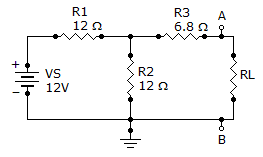# Electrical Engineering - Circuit Theorems and Conversions

### Exercise :: Circuit Theorems and Conversions - General Questions

16.

An 18 V source has an internal resistance of 70. If a load resistance of 33is connected to the voltage source, the load power, PL, is

 A. 0 W B. 1 W C. 175 mW D. 18 mW

Explanation:

No answer description available for this question. Let us discuss.

17.

A certain voltage source has the values VS = 30 V and RS = 6. The values for an equivalent current source are

 A. 5 A, 6B. 30 A, 6C. 5 A, 30D. 30 A, 5Explanation:

No answer description available for this question. Let us discuss.

18.

A 120load is connected across an ideal voltage source with VS = 12 V. The voltage across the load

 A. is 0 V B. is 12 V C. is 120 V D. cannot be determined

Explanation:

No answer description available for this question. Let us discuss.

19.

Find the Norton circuit, that is, IIN and RN, for the circuit given below.A. 478 mA, 12.8B. 750 mA, 12.8C. 478 mA, 6.8D. 750 mA, 6.8Explanation:

No answer description available for this question. Let us discuss.

20.

A 120load is connected across a voltage source with VS = 12 V and RS = 8. The voltage across the load is

 A. 11.25 V B. 0 V C. 12 V D. 1.13 V